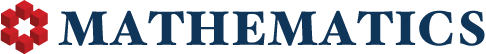## User's Total Reputation Distribution

0

(click on this box to dismiss)Q&A for people studying math at any level and professionals in related fields

``````/* http://stackoverflow.com/a/233223/3184351 */
select u.range as [Reputation Less Than], count(*) as [Number of Users]
from (
select case
when Reputation between 1 and 4 then '4'
when Reputation between 5 and 9 then '9'
when Reputation between 10 and 14 then '14'
when Reputation between 15 and 19 then '19'
when Reputation between 20 and 49 then '49'
when Reputation between 50 and 74 then '74'
when Reputation between 75 and 99 then '99'
when Reputation between 100 and 124 then '124'
when Reputation between 125 and 249 then '249'
when Reputation between 250 and 499 then '499'
when Reputation between 500 and 999 then '999'
when Reputation between 1000 and 1999 then '1999'
when Reputation between 2000 and 2499 then '2499'
when Reputation between 2500 and 2999 then '2999'
when Reputation between 3000 and 4999 then '4999'
when Reputation between 5000 and 9999 then '9999'
when Reputation between 10000 and 14999 then '14999'
when Reputation between 15000 and 19999 then '19999'
when Reputation between 20000 and 24999 then '24999'
else '25000+' end as range
from Users) u
group by u.range``````

### Enter Parameters

Options:
-Hold tight while we fetch your results
:records returned in :time ms:cached